Approximation Ratio for Maximum Edge Disjoint Paths problem

 Importance: Medium ✭✭
 Author(s): Bentz, Cedric
 Subject: Graph Theory
 Keywords: approximation algorithms Disjoint paths planar graph polynomial algorithm
 Posted by: jcmeyer on: April 18th, 2010
Conjecture   Can the approximation ratio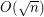be improved for the Maximum Edge Disjoint Paths problem (MaxEDP) in planar graphs or can an inapproximability result stronger than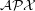-hardness?

Assume a flow graph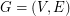withvertices andedges. Each edge has a capacity function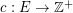(Flow network). The graph contains a listof terminal vertices called sources (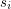) and sinks (). Each pair (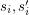) defines a net or commodity.

A Multiflow is a way of routing commodities from their sources to the respective sinks while ensuring that the flow of each commodity is conserved at each non-terminal vertex and that the sum of the flows of all commodities through an edge does not exceed the capacity of the edge.

The Maximum Integer Multiflow problem (MaxIMF) seeks to maximize the number of flow units routed between the nets in the graph. The Maximum Edge Disjoint Paths (MaxEDP) problem seeks to find the maximum number of disjoint paths between the sources and sinks. When the capacities for all edges are set to one, MaxIMF simplifies into the MaxEDP problem.

Bentz provides an algorithm to find the MaxEDP with a proven approximation ratio (Approximation and integrality gap) of. Can the approximation ratio be improved for MaxEDP in planar graphs, or can an inapproximability result stronger than-hardness be proved for this problem? And what about the general graphs?

Bibliography

Cédric Bentz, Edge disjoint paths and max integral muliflow/min multicut theorems in planar graphs, Electronic Notes in Discrete Mathematics 22 (2005), 55–60

* indicates original appearance(s) of problem.